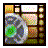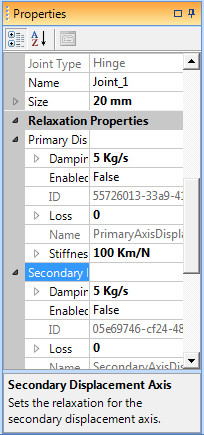# Joint Relaxation## Watch the video tutorial on using constraint relaxation!Joint Relaxation Properties

Joint Relaxation allows you to have direct control of the stiffness, damping, and loss of any of the constraint equations for an axis of a joint. By default relaxation is not enabled for any of axis of a joint so that it uses the default settings for the constraint equations. These default settings are quite stiff and damped. When you select any joint type in the project workspace you will see a "Relaxation Properties" section in the property grid for that joint. Within that section there will be several linear and angular constraint properties listed. The exact number of constraints listed depends on the type of joint you have selected. For example, a hinge joint will have more constraints than a ball-socket joint because he ball-socket has more degrees of freedom available. The number and type of constraints that are available to be edited are specific to the joint type selected.

For each joint constraint listed there are several properties that can be controlled. The first is the Enabled state. This is set to false by default, stating that no relaxation is enabled for this constraint. To turn relaxation on you need to change this to true. You can enable/disable any combination of constraint equations that you want. The next parameter is the Stiffness. This determines the stiffness for the spring used to model that constraint equation. If you would like that constraint to be a little looser then you would need to decrease the stiffness. The next parameter is the damping. This determines how much damping to apply to the movement of around this constraint. Finally, you can also specify the kinetic loss coefficient for this joint constraint equation.

## Joint Relaxation Properties

Enabled
Determines if relaxation is enabled for this joint constraint equation, or if the default values will be used.
Default value: False
Acceptable range:True or False

Stiffness
Determines the stiffness of the spring used to model the constraint.
Default value: 100 Km/N
Acceptable range:Any value greater than 0

Damping
Determines the damping of the spring used to model the constraint.
Default value: 5 Kg/s
Acceptable range:Any value greater than 0

Loss
Determines the coefficient of kinetic loss for the spring.
Default value: 0
Acceptable range:Any value greater than or equal to 0